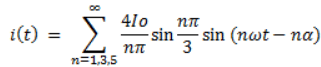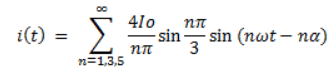# Test: Advance Three Phase Converters

Test Description

## 10 Questions MCQ Test Power Electronics | Test: Advance Three Phase Converters

Test: Advance Three Phase Converters for Electrical Engineering (EE) 2023 is part of Power Electronics preparation. The Test: Advance Three Phase Converters questions and answers have been prepared according to the Electrical Engineering (EE) exam syllabus.The Test: Advance Three Phase Converters MCQs are made for Electrical Engineering (EE) 2023 Exam. Find important definitions, questions, notes, meanings, examples, exercises, MCQs and online tests for Test: Advance Three Phase Converters below.
Solutions of Test: Advance Three Phase Converters questions in English are available as part of our Power Electronics for Electrical Engineering (EE) & Test: Advance Three Phase Converters solutions in Hindi for Power Electronics course. Download more important topics, notes, lectures and mock test series for Electrical Engineering (EE) Exam by signing up for free. Attempt Test: Advance Three Phase Converters | 10 questions in 10 minutes | Mock test for Electrical Engineering (EE) preparation | Free important questions MCQ to study Power Electronics for Electrical Engineering (EE) Exam | Download free PDF with solutions
 1 Crore+ students have signed up on EduRev. Have you?
Test: Advance Three Phase Converters - Question 1

### In a 3 phase M-6 controlled converter for continuous conduction mode, each SCR conducts for __________ per cycle.

Detailed Solution for Test: Advance Three Phase Converters - Question 1

Each SCR conducts for 60° or 2π/6 radians. 6 x 60 = 360°.

Test: Advance Three Phase Converters - Question 2

### In a 3 phase, 12-pulse controlled converter for continuous conduction mode, each SCR conducts for __________ per cycle.

Detailed Solution for Test: Advance Three Phase Converters - Question 2

Each SCR conducts for 30° or π/6 radians. 12 x 30 = 360°.

Test: Advance Three Phase Converters - Question 3

### A 3-phase full converter delivers a ripple free load current of 10 A with a firing angle delay of 45°. The input voltage is 3-phase, 400 V, 50 Hz. The source current is given by the following relation.Find the fundamental component of the source current amplitude.

Detailed Solution for Test: Advance Three Phase Converters - Question 3

Put n = 1 (fundamental component) and the rest of the given values in the above given equation.

Test: Advance Three Phase Converters - Question 4

A 3-phase full converter delivers a ripple free load current of 10 A with a firing angle delay of 45°. Find the DF.

Detailed Solution for Test: Advance Three Phase Converters - Question 4

DF = cosα = cos45 = 0.707
Note that ripple free current does not mean that DF = 0. It means the current is continues in magnitude and direction, current will always contain harmonics (distortions) whether it is rippled or ripple free.

Test: Advance Three Phase Converters - Question 5

What is the relationship between DF, CDF and PF?

Detailed Solution for Test: Advance Three Phase Converters - Question 5

PF (power factor) = (distortion factor) x (current distortion factor).

Test: Advance Three Phase Converters - Question 6

The commutation period when both incoming and outgoing SCRs are conducting due to source inductance is called as the

Detailed Solution for Test: Advance Three Phase Converters - Question 6

Due to source inductance, SCRs cannot start and stop conducting immediately, hence a time occurs when both incoming and outgoing SCRs are conducting together. This delay is called as commutation angle or overlap period (μ).

Test: Advance Three Phase Converters - Question 7

A 3-phase full converter delivers a ripple free load current of 10 A with a firing angle delay of 45°. The input voltage is 3-phase, 400 V, 50 Hz. The source current is given by the following relation.Find the value of 2nd harmonic source current amplitutue.

Detailed Solution for Test: Advance Three Phase Converters - Question 7

2nd harmonics are absent in a 3-phase full converter, it has only odd number of harmonics i.e. 3rd, 5th etc.

Test: Advance Three Phase Converters - Question 8

In a p-pulse converter, each SCR conducts for (per cycle)

Detailed Solution for Test: Advance Three Phase Converters - Question 8

In a p-pulse controlled converter, each device conducts for p radians. p-pulse converter would have p devices. Hence, 2π/p x p = 2π = 360°.

Test: Advance Three Phase Converters - Question 9

Which of the below mentioned converter can operate in both 3-pulse and 6-pulse modes?

Detailed Solution for Test: Advance Three Phase Converters - Question 9

Semi-converters can operate in 6-pulse modes gating the SCRs at appropriate intervals.

Test: Advance Three Phase Converters - Question 10

A M-6 controlled converter or 6-pulse half-wave controlled converter is obtained by using a transformer having

Detailed Solution for Test: Advance Three Phase Converters - Question 10

M-6 requires a transformer having a delta connected primary and a double star connected secondary such that 6 SCRs are connected to it on the secondary side.

## Power Electronics

5 videos|39 docs|63 tests
 Use Code STAYHOME200 and get INR 200 additional OFF Use Coupon Code